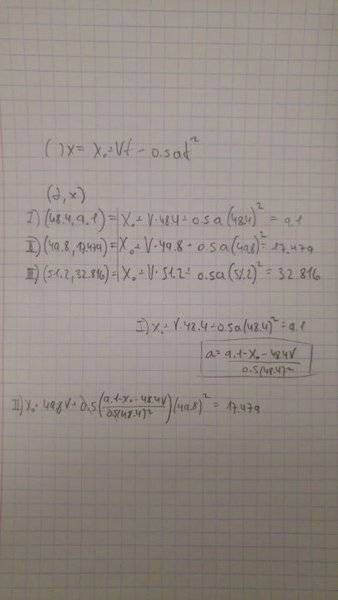# Finding acceleration given position and time

## Homework Statement

An object is moving in a straight line with a constant acceleration. Its position is measured at three different times, as shown in the table below.
Time (s) | Position, (m)
48.40 | 9.100
49.80 | 17.479
51.20 | 32.816
Calculate the magnitude of the acceleration at t=49.80 s.

## Homework Equations

V(t) = V=at
r(t)=r+v0t+0.5at2
a=ΔV / Δt

## The Attempt at a SolutionI started making a 3 equations system, but this got way more complex than I think it should, and way more complicated than we did in class... Is this even the right direction at solving this?

EDIT: sorry for the hassle, solved the question.

Last edited: